## Index

 STA & SI Chapter1 Chapter2 Chapter3 Chapter4 Chapter5 Chapter6 Chapter7 Chapter8 Introduction Static Timing Analysis Clock Advance STA Signal Integrity EDA Tools Timing Models Other Topics

 Extraction & DFM Chapter1 Chapter2 Chapter3 Chapter4 Chapter5 Chapter6 Introduction Parasitic Interconnect Corner (RC Corner) Manufacturing Effects and Their Modeling Dielectric Layer Process Variation Other Topic

## Sunday, September 30, 2012

### Maximum Clock Frequency : Static Timing Analysis (STA) basic (Part 5b)

 STA & SI:: Chapter 2: Static Timing Analysis 2.1 2.2 2.3a 2.3b 2.3c 2.4a Timing Paths Time Borrowing Basic Concept Of Setup-Hold Basic Concept of Setup-Hold Violation Examples:S-H Time/Violation Timing Path Delay 2.4b 2.4c 2.5a 2.5b 2.6a 2.6b Interconnect Delay Models Delay - Wire Load Model Maximum Clock Frequency Calculate “Max Clock Freq”-Examples Fix Setup-Hold Violation-1 Fix Setup-Hold Violation-2 2.6c 2.7a 2.7b 2.7c 2.8 Fix Setup-Hold Violation-3 Incr/Decr Delay Method-1 Incr/Decr Delay Method-2 Incr/Decr Delay Method-3 10 ways to fix Setup-Hold Violation.

Static Timing analysis is divided into several parts:

### Example 1: Multiple FF’s Sequential Circuit

In a typical sequential circuit design there are often millions of flip-flop to flip-flop paths that need to be considered in calculating the maximum clock frequency. This frequency must be determined by locating the longest path among all the flip-flop paths in the circuit. Consider the following circuit.

There are three flip-flop to flip-flop paths (flop A to flop B, flop A to flop C, flop B to flop C). Using an approach similar to whatever I have explained in the last section, the delay along all three paths are:

• TAB = tClk−Q(A) + ts(B) = 9 ns + 2 ns = 11 ns
• TAC = tClk−Q(A) + tpd(Z) + ts(C) = 9 ns + 4 ns + 2 ns = 15 ns
• TBC = tClk−Q(B) + tpd(Z) + ts(C) = 10 ns + 4 ns + 2 ns = 16 ns
Since the TBC is the largest of the path delays, the minimum clock period for the circuit is Tmin = 16ns and the maximum clock frequency is 1/Tmin = 62.5 MHz.

### Example 2: Circuit with min and max delay Specification

Let’s consider following circuit. Now this circuit is similar to the normal FF circuitry, only differences are

• Every specification has 2 values (Min and Max).
• There is a combinational circuit in the clock path also.
Note: if you are wondering why there are min and max value (or like from where these values are coming, then you have to refer another blog).

Now let’s understand the flow/circuit once again.
• Every interconnect wire also has some delay, so you can see clock CLK will take some time to reach the clock pin of the FF1.
• That’s means with reference to original clock edge (let’s assume at 0ns), clock edge will take minimum 1ns and maximum 2ns to reach the clock pin of the FF1.
• So in the similar fashion, if we will calculate the total minimum delay and maximum delay.
• In data path : max delay = (2+11+2+9+2)ns=26ns
• In data path : min delay = (1+9+1+6+1)ns=18ns
• In clock path: max delay= (3+9+3)ns=15ns
• In clock path : min delay = (2+5+2)ns=9ns
• In the last 2 example, there were no delays in the clock path, so it was easy to figure out the minimum clock period. But in this example we have to consider the delay in the clock path also.
• So for minimum clock period, we just want to make sure that at FF2, data should be present at least “tsetup” time before positive clock edge (if it’s a positive edged triggered flipflop) at the FF2.
• So Clock edge can reach at the FF2 after 9ns/15ns (min/max) with the reference of original clock edge.
• And data will take time 18ns/26ns (min/max) with the reference of original clock edge.
• So clock period in all the 4 combinations are
• Clock period (T1)= (Max data path delay)-(max clock path delay)+tsetup=26-15+4=15ns
• Clock period (T2)= (Min data path delay)-(max clock path delay)+tsetup=18-15+4=7ns
• Clock period (T3)= (Max data path delay)-(min clock path delay)+tsetup=26-9+4=21ns
• Clock period (T4)= (Min data path delay)-(min clock path delay)+tsetup=18-9+4=11ns
• Since we want that this circuit should work in the entire scenario (all combination of data and clock path delay), so we have to calculate the period on the basis of that.
• Now if you will see all the above clock period, you can easily figure out that if the clock period is less than 21ns, then either one or all of the scenarios/cases/combinations fail.
• So we can easily conclude that for working of the entire circuit properly
• Minimum Clock Period = Clock period (T3) = (Max data path delay)-(min clock path delay)+tsetup=26-9+4=21ns
So in general:
Minimum Clock Period = (Max data path delay)-(min clock path delay) + tsetup

And "Maximum Clock Frequency = 1/(Min Clock Period)”

### Example 3: Circuit with multiple Combinational paths between 2 FFs:

Now same scenario is with this example. I am not going to explain much in detail. Just it’s like that if you have multiple paths in between the 2-flipflops, then as we have done in previous examples, please calculate the delays.
Then calculate the time period and see which one is satisfying all the condition. Or directly I can say that we can calculate the Clock period on the bases of the delay of that path which has big number.
Min Clock Time Period = Tclk-q (of UFF1) + max(delay of Path1,delay of Path2) +Tsetup (of UFF3)

### Example 4: Circuit with Different kind of Timing paths:

Since I have mentioned that it has different kind of timing path, so you should know about the timing paths. For that you can refer the (Post link) post. After reading the Timing path, you can easily figure out that in the above circuit there are 4 types of data paths and 2 clock paths

Data path:

1. Register to register Path
• U2 -> U3 ->U1 (Delay=5+8=13ns)
• U1 -> U4 -> U2 ( Delay=5+7=12ns)
2. Input pin/port to Register(flip-flop)
• U7 ->  U4 -> U2 ( Delay=1+7=8ns)
• U7 -> U3 -> U1 ( Delay=1+8=9ns)
3. Input pin/port to Output pin/port
• U7 -> U5 -> U6 (Delay=1+9+6=16ns)
4. Register (flip-flop) to Output pin/port
• U1 -> U5 -> U6 (Delay=5+9+6=20ns)
• U2 -> U5 -> U6 (Delay=5+9+6=20ns)

Clock path:

• U8 -> U1 (Delay = 2ns)
• U8 -> U2 (Delay =2ns)

Now few important points- This is not a full chip circuit. In general, recommendation is that you use registers at every input and output port. But for the time being, we will discuss this circuit, considering this as full chip circuit. And you will how much analysis you have to do in this case. Next example, I will add the FFs (registers) at input and output port and then you come to know the difference.

Now let’s Study this circuit in more details.

• In this circuit, we have to do the analysis in such a way that if we will apply an input at Port A, then how much time it will take to reach at output Port Y. It will help us to find out the time period of clock.
• Output pin Y is connected with a 3input NAND gate. So if we want a stable out at Y, we have to make sure that all 3 Inputs of NAND gate should have stable data.
• One input of NAND gate is connected with Input pin A with the help of U7.
• Time take by data to reach NAND gate is 1ns (gate delay of U7)
• Second input pin of NAND gate is connected with output pin Q of Flip flop U2.
• Time take by data which is present at input D of FF –U2 to reach NAND gate:
• 2ns(delay of U8)+5ns(Tc2q of FF U2)=7ns
• Third input pin of NAND gate is connected with the output pin Q of Flip Flop U1.
• Time take by data which is present at input D of FF –U2 to reach NAND gate:
• 2ns(delay of U8)+5ns(Tc2q of FF U1)=7ns
Note:
• I know you may have doubt that why delay of U8 comes in picture.
• With reference to the clock edge at CLK pin, we can receive the data at NAND pin after 7ns only (Don’t ask me- why we can’t take reference in negative?)
• May be you can ask why we haven’t consider the setup time of FF in this calculation.
• If in place of NAND gate, any FF would there then we will consider the setup. We never consider the setup and Tc2q (Clk-2-Q) values of same FF in the delay calculation at the same time. Because when we are considering Clk-2-Q delay, we assume that Data is already present at input Pin D of the FF.

So Time required for the data to transfer from input (A) to output (Y) Pin is the maximum of:

Pin2Pin Delay = U7+U5+U6 = 1+9+6=16ns
Clk2Out (through U1) delay = U8 +U1+U5+U6=2+5+9+6=22ns
Clk2Out (through U2) delay = U8 +U2+U5+U6=2+5+9+6=22ns.

So out of this Clk2Out Delay is Maximum.

From the above Study, you can conclude that data can be stable after 7ns at the NAND gate and maximum delay is 22ns. And you can also assume that this much data is sufficient for calculating the Max Clock Frequency or Minimum Time Period. But that’s not the case. Still our analysis is half done in calculating the Max-clock-frequency.

As we have done in our previous example, we have to consider the path between 2 flip-flops also. So the paths are:
• From U1 to U2 (Reg1Reg2)
• Path delay= 2ns (Delay of U8) + 5ns (Tclk2Q of U1)+7ns (Delay of U4)+3ns (Setup of U2) – 2ns (Delay of U8)=17ns-2ns=15ns
• From U2 to U1 (Reg2Reg1)
• Path delay = 2ns (Delay of U8) + Tclk2Q of U2 (5ns) + Delay of U3 (8ns) + setup of U1 (3ns) – Delay of U8 (2ns) =18ns -2ns = 16ns.

Note:

• I am sure you will ask why did I subtract “Delay of U8” from the above calculation :) because Delay of U8 is common to both the launch and capture path (In case you want to know what’s Launch and capture path please follow this post).  So we are not supposed to add this delay in our calculation. But just to make it clear, I have added as per the previous logic and then subtracted it to make it clear.

So now if you want to calculate the maximum clock frequency then you have to consider all the delay which we have discussed above.

So
Max Clock Freq = 1/ Max (Reg1Reg2, Reg2Reg1, Clk2Out_1, Clk2Out_2, Pin2Pin)
= 1/ Max (15, 16, 22, 22, 16)
=1/22 =45.5MHz

### Example 5: Circuit with Different kind of Timing paths with Register at Input and output ports:

In this example, we have just added 2 FFs U8 at Input pin and U9 at output pin. Now for this circuit, if we want to calculate the max clock frequency then it’s similar to example 1.
There are 7 Flip flop to flipflop paths

1. U8 -> U4 -> U2
• Delay = 5ns+7ns+3ns=15ns
2. U8 -> U3 -> U1
• Delay = 5ns+8ns+3ns=16ns
3. U8 -> U5 -> U9
• Delay = 5ns+9ns+3ns=17ns
4. U1 -> U4 -> U2
• Delay = 5ns +7ns +3ns = 15ns
5. U1 -> U5 -> U9
• Delay= 5ns+9ns+3ns=17ns
6. U2 -> U5 -> U9
• Delay=5ns+9ns+3ns=17ns
7. U2 -> U3 -> U1
• Delay=5ns+8ns+3ns=16ns

Since the maximum path delay is 17ns,
The Minimum clock period for the circuit should be Tmin = 17 ns
And the Maximum clock frequency is 1/Tmin = 58.8 MHz.

1.Awesome stuff. Will you also talk a little about multi-cycle paths?

1.Sure - I will do in next few articles.

2.Hello Sir,

Big fan of yours blog.
I have a query.
In Example 3(Circuit with multiple Combinational paths between 2 FFs), u ve mentioned-
Min Clock Time Period = Tclk-q (of UFF1) + max(delay of Path1,delay of Path2) –Tsetup (of UFF3).

is this correct? If yes, how come?

1.Hi,
The above mentioned statement is correct. For explanation, I will prefer you to read the previous part of this series and the first 2 example.
Even after that if you have any doubt, just let me know.

3.Hi,
I still can't understand Example 3. Means I thought the min clock period should be Delay from Clk to Q of f/f1 + max(Td delay of path1, Td delay of path2) + Ts of f/f3; but why there is -Ts of f/f3? Normally we should add the setup time to get the min clock period, but here you are subtracting. Can you please explain it, I am getting confused.

1.Means data which reaches the D of f/f3, should get stable before the Ts time of the next clock edge. So, Ts should get added to complete the clock period. But why u are subtracting. Please explain it little. I read the blog, but didn't understood.

4.Sir,
even I have same doubt about example3. plz explain sir...

1.Hi,

5.Hi,

For calculationg the max frequency you have used
Max Clock Freq = 1/ Max (Reg1Reg2, Reg2Reg1, Clk2Out_1, Clk2Out_2, Pin2Pin)

I think Max clock frequency = 1/Min (Reg1Reg2, Reg2Reg1, Clk2Out_1, Clk2Out_2, Pin2Pin)

Max clock frequency = 1/Min(T). Please correct me if i am wrong

1.:) .. you know, I was expecting some thing similar to this from long time... :)
for the time being - dnt think about max and min value of any parameter.

Now Clock frequency = 1/Time_period
and Time period depends on the delay of the circuit.
Now if in your circuit - there are 2 paths between FFs - one having 1sec delay and other 1.5s and you want that data should reach from launching to capturing FF with in one clock cycle and it should satisfy both the condition (both the delays), then which one do you choose ?
If I am correct, you have to decide the delay as per the delay path of 1.5s. Means Minimum Time period should be 1.5s. You can have more then this but 1.5s should be the minimum value.
and then the frequency will be f=1/1.5.
Now if Time period is more then this, then frequency will be less then 1/1.5 and that the reason we are saying that f=1/1.5 is the Maximum frequency.
So you are right max_frequency=1/min_T.
but as you have seen that Min_T depends on the Maximum delay in the circuit.
I hope you got the reason of choosing Maximum delay out of all the possible delay values present between the 2 FFs. Reason is - we want to satisfy all the delay path.

Let me know if still you have any follow on question.

2.6.Hello sir, In the example 2 while calculating maximum frequency you have to subtract skew of destination filp-flop w.r.to source flip-flop,

Therefore, Tmin=11+2+9+2+4-((2+5+2)-1)=20 ns

Isn't it?
am i correct or wrong?

1.looks like your calculation is little bit incorrect. Just check once again.

2.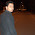Tmin=2+11+2+9+2+4 - ((2+5+2)-1)=22 ns is it correct?

7.Hi,
For example 3: Min clock period calculation is written as,
Min Clock Time Period = Tclk-q (of UFF1) + max(delay of Path1,delay of Path2) –Tsetup (of UFF3)

But i feel, Instead of subtracting Tsetup (of UFF3)(-) it should have added(+) in the above equation.
Correct me if i am wrong.

1.Correct ... and I have corrected also. thanks for correction

8.Hi,
In example 2,
"In data path : min delay = (1+9+1+9+1)ns=21ns".
Is it "(1+9+1+6+1)ns=18ns ?
Thanks.

1.100% correct. I have corrected also in my post. thanks for correction.

9.Hi,
In example 3 it is Min Clock Time Period = Tclk-q (of UFF1) + max(delay of Path1,delay of Path2) + Tsetup (of UFF3)

1.Correct ... and I have corrected also. thanks for correction

10.hi,
when you calculate Max Clock Freq in example 4, why don't you consider the delay between input pin and flip-flop? thanks.

11.sir,
Here in calculation of maximum frequency why are you not considering the input to reg delay. please explain?

12.I have the same question as venkata krishna, why you don't consider the input to register delay, when calculating the max. clock frequency. Thanks.

1.The reason we are not considering in this example ... you have freedom to apply the input at any time before the first positive clock pulse. Even if you want to capture the second set of input data at any of the flipflop ... you don't have any restriction to apply the data at input port. so on what base you will define a relationship between clock frequency and "input to flop delay".

Let me know, if still you have confusion ...

2.I am not sure whether I get your point. As you also consider the input-to-output and register-to-output delay in the clock frequency calculation, you are basically assuming the inputs and the outputs are sampled by the clock (probably with a sampling offset with respect to the rising edge of the clock, assuming an ideal clock net). As long as the data are sampled, there is a relationship between input/output and the clock. Am I wrong?

3.I was thinking to raise the point of input-to-output path in my reply but somehow I deleted. Point of including that one is -- there is a NAND gate which has 3 input. Out of 3 - 2 are coming from a flop and the signal which is coming from flop has dependence on the clock .. And I have mentioned that
"Output pin Y is connected with a 3input NAND gate. So if we want a stable out at Y, we have to make sure that all 3 Inputs of NAND gate should have stable data. "
And that's the reason we have to consider this input-to-output path.

Lets suppose this is just a simple wire .. and it has no relationship with the clock , then there was no need of this path in our calculation.

You are right - if we are sampling the data then there is a relationship but whether that sampling is going to effect you clock frequency or not -- that's the question. Let us suppose that I am asking you to capture a train (any train).. then you can start from your home at any time and you will not bother about the time taken to reach railway station but the moment I mention that you have to capture a train at 10PM , then you will start calculating the time taken to travel and margin and so many things.

So in our case , you can apply the Input at any time and I have not mentioned anywhere that first flipflop should capture the data at time = 2ns or 1 ns or 0ns or anyother time. And that's the reason I have not taken the input to flop into calculation.

I hope you got my point. Let me know if still you have confusion.

4.Now I get your point. Many thanks for your detailed explanation. I like your blog very much and learned a lot.

5.Welcome ... and thanks for appreciating.

6.hello sir
thanq
I have gone through previous blogs

I have similar doubt that why we consider I/p to o/p because in the case similar, input to reg. would also be considered because input of U3 also depend on FF U2 o/p (depends on clock) so data must be stable at d for next clock.
that is I am confused weather to consider - input to register also (as asked above) and combinational ckt (only wire)

and secondly the delay from Reg to o/p which contains only combinational circuit (identical to wire delay) should NOT be considered , i.e. (9+6) should not be included in 22ns that is in clock to out only u8-u5 should be there, after that ff will lock it (or get stable) and it will travel to o/p.
that is register to output(o/p) should it only be considered uptil ff o/p excluding combinational circuit.
sir plz explain I am confused

13.I have started with the blogs and they are awesome and very informative. I have a doubt in example 2. You have used-
Minimum Clock Period = Clock period (T3) = (Max data path delay)-(min clock path delay)+tsetup

Please tell why we are subtracting min clock path delays, because, if we are calculating clock period then we should accommodate all the delays?

1.we are calculating the Minimum required Clock period so that our circuit can work.. another thing - these 2 path are different. Clock travel through clock path, and it will take some time to reach the capture FF. Now Data travelling also taking some to reach capture FF. So now if you want to calculate the effective time difference, you have to subtract Time taken by clock to Capture FF from the time taken by data to reach capture FF.
Now this effective time difference is going to help in deciding the Minimum clock period.

I hope you get my point. If not try to visualize this and if still have issue, let me know.

2.i have the same doubt and i cant able to visualize this....i have doubt that we want to cal min clock period,,i think for that data path calculation is sufficient...it gives total delay so why we consider clk path also?

3.You are partially right .. that Data path delay is sufficient only when Clock path is Ideal means no delay.
You want to capture a data at Capture Flipflop... in the data path there is a delay of 10ns. That means you can't capture the data before that (10ns) (if there is no setup value). And as per your understanding - it's sufficient. So as per your understanding Minimum Clock Period should be 10ns. Am I right ?
Think, what if Clock path has a delay of 2ns. Since Clock path already have a delay, so as per your calculation, Clock period : 10nm and delay of 2ns: Means total 12ns after Clock will reach to Capture Flipflop. But minimum Time of 10ns is sufficient.

Now in such case we can reduce the Clock period to 8nm. Now 8+2=10.

I think, Now you can easily visualize that why clock path is also important for Calculating Minimum Clock Period.

Let me know if you still have doubt.

14.why have we not considered the clk to out delay in last example?
Though its only 15 ns (less than maximum) but still should be mentioned.
am I right that we need to consider clk to out delay or not?

1.yaa..i am also thinking so..

15.Hi, In Example 2: FF1 to FF2 is Register to Register Path.
For Calculating Reg to Reg path, we considered Ts of Flop 2 in Examples.
why You here Not added the Ts in calculations??
So in the similar fashion, if we will calculate the total minimum delay and maximum delay.

In data path : max delay = (2+11+2+9+2)ns=26ns
In data path : min delay = (1+9+1+6+1)ns=18ns
In clock path: max delay= (3+9+3)ns=15ns
In clock path : min delay = (2+5+2)ns=9ns

Minimum Clock Period = Clock period (T3) = (Max data path delay)-(min clock path delay)+tsetup=26-9+4=21ns

MY Calculations:
In data path : max delay = (2+11+2+9+2+4[ts of FF2])ns=30ns
In data path : min delay = (1+9+1+6+1+4[ts of FF2])ns=22ns
In clock path: max delay= (3+9+3+4[ts of FF2])ns=19ns
In clock path : min delay = (2+5+2+4[ts of FF2])ns=13ns

Minimum Clock Period = Clock period (T3) = 30-9=21ns

My Question is as per Technical Terminology, minimum delay and maximum delay means we should not add the Ts of 2nd Flop????

Thanks for the Article.

1.Hi First of all .. you can't add setup in both places (clock path and data path)... so what ever you have done is not correct.
In the above example you can see that later on while calculating the time period , setup time is considered..

Clock period (T1)= (Max data path delay)-(max clock path delay)+tsetup=26-15+4=15ns
Clock period (T2)= (Min data path delay)-(max clock path delay)+tsetup=18-15+4=7ns
Clock period (T3)= (Max data path delay)-(min clock path delay)+tsetup=26-9+4=21ns
Clock period (T4)= (Min data path delay)-(min clock path delay)+tsetup=18-9+4=11ns

Now if you are talking about the data path only - you can do the calculation as per that also .. means

In data path : max delay = (2+11+2+9+2+4[ts of FF2])ns=30ns
In data path : min delay = (1+9+1+6+1+4[ts of FF2])ns=22ns
In clock path: max delay= (3+9+3)ns=15ns
In clock path : min delay = (2+5+2)ns=9ns

and now the Clock period (T3) will be 30-9=21ns.

So you can calculate in this way also.
Regarding the Technical Terminology - frankly speaking - I don't care because for me concept is important. and no one is going to ask definition.
As such when I am saying min or max delay of Net then it don't consider the setup and hold time , when you saying min and max of cell - then only cell delay is considered and when you are saying min and max of wire then only wire delay is considered.
If you are saying that min and max of data path then you may include setup and hold time. But I always consider them separate - it's easy to remember.

BTW nice observation and good analysis.

16.Example 3: Circuit with multiple Combinational paths between 2 FFs:
Min Clock Time Period = Tclk-q (of UFF1) + max(delay of Path1,delay of Path2) +Tsetup (of UFF3)

But there was 3 paths available.Path1 & Path 2 was Defined in Diagram.
Path 3: UFF1 -> UNAND0 -> UBUF2 -> UNAND6 -> UFF3.

then

Min Clock Time Period = Tclk-q (of UFF1) + max(delay of Path1 or delay of Path2 or delay of Path3 ) +Tsetup (of UFF3)

Right ??

1.Yes You are right ... The reason I didn't capture .. because we know that path 3 delay will be always greater then other one (because of missing one GATE there... )

17.HI, In Example 4 Data paths & Clock paths was Calculated.

Data path:

1. Register to register Path
o U2 -> U3 ->U1 (Delay=5+8=13ns)
o U1 -> U4 -> U2 ( Delay=5+7=12ns)
2. Input pin/port to Register(flip-flop)
o U7 -> U4 -> U2 ( Delay=1+7=8ns)
o U7 -> U3 -> U1 ( Delay=1+8=9ns)
3. Input pin/port to Output pin/port
o U7 -> U5 -> U6 (Delay=1+9+6=16ns)
4. Register (flip-flop) to Output pin/port
o U1 -> U5 -> U6 (Delay=5+9+6=20ns)
o U2 -> U5 -> U6 (Delay=5+9+6=20ns)
Clock path:

• U8 -> U1 (Delay = 2ns)
• U8 -> U2 (Delay =2ns)

And Calculated Time required for the data to transfer from input (A) to output (Y) Pin is the maximum of:

Pin2Pin Delay = U7+U5+U6 = 1+9+6=16ns
Clk2Out (through U1) delay = U8 +U1+U5+U6=2+5+9+6=22ns
Clk2Out (through U2) delay = U8 +U2+U5+U6=2+5+9+6=22ns.
So out of this Clk2Out Delay is Maximum.

Is it Mandatory to calculate ??
Normally we will consider the paths: 1. i/p to o/p 2.i/p to Reg 3.Reg to Reg 4. Reg to o/p for Timing Calculations.

If It is Mandatory to calculate i/p to o/p & Clk2Q to o/p then
Q1). Explain me If suppose Example 2 has one more Flop like FF3, Same delay between FF2 to FF3 & Same Clock Delay between FF2 to FF3
Are we going calculate the i/p of FF1 to o/p of FF3 & Clk2Q of FF1 to o/p of FF3 ??? ( I think NO )

You calculated Pin2Pin Delay, Clk2Out (through U1) delay & Clk2Out (through U2) delay, because of getting o/p Y, 3 multiple i/p's required for the AND(U5) gate ???
Pin2Pin Delay = U7+U5+U6 = 1+9+6=16ns
Clk2Out (through U1) delay = U8 +U1+U5+U6=2+5+9+6=22ns
Clk2Out (through U2) delay = U8 +U2+U5+U6=2+5+9+6=22ns.

Let me Know In what cases, I should calculate Pin2Pin Delay, Clk2Out (through U1) delay & Clk2Out (through U2) delay ???

Q2). Explain me If suppose Example 2 has one more Flop like FF3, Same delay between FF2 to FF3 & Same Clock Delay between FF2 to FF3 But FF1 is @Posedge clk , FF2 is @Negedge clk & FF3 is @Posedge clk

Q3).Explain me If suppose Example 2 has one more Flop like FF3, delay between FF2 to FF3 Tmin=3ns, Tmax=12ns & Clock Delay between FF2 to FF3 Tmin=2ns, Tmax=15ns

Q4). Explain me If suppose Example 2 has one more Flop like FF3, delay between FF2 to FF3 Tmin=3ns, Tmax=12ns & Clock Delay between FF2 to FF3 Tmin=2ns, Tmax=15ns But FF1 is @Posedge clk , FF2 is @Negedge clk & FF3 is @Negedge clk

Q5). In Example 5 is it not required to Calculate Time required for the data to transfer from input (A) to output (Y) Pin is the maximum of:

Pin2Pin Delay = ???
Clk2Out (through U1) delay = ???
Clk2Out (through U2) delay = ???

May be these are lengthy Questions....But I want to Know & Expecting Clear Answers from You......Thanks in Advance.

1.HAHAH ... So many questions ...
CLK2Q is necessary to calculate ... because that's also one type of delay which can effect timing calculation.

If 1 more FF is present in the example 2 and if that's connected to FF1 directly (means no other FF between FF1 and FF3) then we are going to calculate all the Reg-to-reg delay.
Always remember basic concept.

Answer2: if it's positive edge and negative edge, then you can remove/add the effect of that part. So if launched FF is at time 0 and capture FF is at time same clock pulse the negative edge - then you can remove the OFF time of clock while you are calculating the clock requirement.
Means if duty cycle is 50%, then replace min_clock_time_period with 2*min_clock_time_period in above calculation.
But if capture FF is at time 1complet clock pulse + On time of second pulse (means negative edge of second pulse ) then equation will be different.
I hope you get my point.

Answer3 and 4: Do you think practically it's possible.. ???

2.Let me Know one thing....

You calculate Time required for the data to transfer from input (A) to output (Y) Pin is the maximum of:

Pin2Pin Delay = U7+U5+U6 = 1+9+6=16ns
Clk2Out (through U1) delay = U8 +U1+U5+U6=2+5+9+6=22ns
Clk2Out (through U2) delay = U8 +U2+U5+U6=2+5+9+6=22ns.

You calculated Pin2Pin Delay, Clk2Out (through U1) delay & Clk2Out (through U2) delay because the FF1 to o/p & FF2 to o/p paths available??? Right

If the Flops are cascaded one to other flop like FF1 to FF2, FF2 to FF3, FF3 to FF4 ...etc then there won't be above calculations???. Right

Can you explain Q2, Q3, Q4 & Q5 with the calculations Practically here........I am Expecting calculations.....

3.The above Explanation is Correct or NOT ??

18.In Example 4 there was Clock delay (U8) = 2ns for Both Flops U1 & U2.

Q1). For Suppose CK delay (U8) = 2ns common for Both Flops U1,U2 & Extra Ck Delay (U8_Inverter) for Flop U2, then How will calculate Tmin & Fmax ???

1.Then it will be almost similar to example 2 .. use example 2 concept and then try to recalculate ....

2.I am expecting with calculations..... so that If we have any setup & Hold violations, How we can play with the Delay's....

3.hi That's what my point .. please read the different post and then try your self first. Come up with exact questions and what have you tried .. then I can help you.
If I will do every thing for you .. then it will be difficult for you to learn.
I hope you are getting my point.

19.dear Expert,

i just want to know among four types of datapaths, does the pin to pin (input to output) path must necessarily have only combinational block between pins? i mean, can it not have any register or flop in between? (or, will it now be treated as input-to-reg path??)

1.you are right .. The moment it will have a register - it will be treated as input-to reg and reg-to-output paths.

20.hi
i have a small doubt in example 4.
in example 4
with how much frequency input A is changing?
is it same as the clock frequency or not?

1.Here we are not talking about the Frequency by which A is changing. But you are right - The real life it will have a dependency on frequency .. and the moment it will depend on frequency - things will change -- So right now in this example - just consider this as just like that.

21.Great Work. Thanks a lot.

22.Can you please explain last example,5? Why maximum path delay is not 22 same as previous example. Why are we considering only reg to reg delay and not other delays?

23.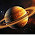Hello sir,

I have a very basic question on setup and hold time.
Suppose we have a critical path which consists of 4 ff and combo delays in between them.
My question is :

Can we have setup and hold violation on the same path ?
Can we have setup and hold violations on the same flop ?

Please let me know on this.

REgards
Tanvi

24.Hi. In Example 4, why have you not included 2ns delay from the clock source?
I think, it should be Register to Register data path= 2ns +5ns+ 8ns= 15ns (U2->U3->U1).

25.Hi, I believe external I/O delays will also limit the max operating frequency of our circuit. Can you confirm my understanding please ?

It will be great if you can add an example circuit with input delay and output delay. Other than that I believe you have covered all other basic circuits.

Thanks

1.Hi, I have also noticed that in Example4 in max operating frequency calculation only reg2reg, reg2out and pin2pin delays are considered. Why are we not considering in2reg path also (input to register) ?

26.what is max freq when inverter is placed instead of buffer with 50% dutycyle is it remains same or it will change?

27.for max path delay do we take path from flipflop to flipflop or clock to output?? in example 5 only R2R paths are calculated and c2q not considered and in ex 4 clock to output is considered!

28.IN Q5. why are we not considering the clk to output delay which will be 27ns (the minimum clock period required)? please reply.

29.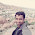PD Clock- Output (min) = Rpd (min) + Gpd (min)

PD Clock- Output (max) = Rpd (max) + Gpd (max)

MCLK = 1/ TMIN
Where TMIN = Fpd (MAX) + RSETUP + Rpd (MAX)

In these above equation What is meant by Rpd,Gpd and Fpd??

30.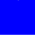in example 4 for calculating max freq we should find out min clock period and that min clock period should not violate setup condition in any other flop of circuit

in this example two paths are there b/w flops
1st U2to U1 Tclk>=16ns
2nd U1 to U2 Tclk>=15ns

for max freq we should take min clock period(tclk >=15ns) but this will violate setup time between U2 and U1(15>=16)
therefore Tclk>=16ns
fclk(max)=62.5MHz
am i right?

31.Your article is detailed, thanks to it I solved the problem I am entangled. I will regularly follow your writers and visit this site daily.

1.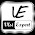Thanks for appreciating.

32.Your article is awesome! How long does it take to complete this article? I have read through other blogs, but they are cumbersome and confusing. I hope you continue to have such quality articles to share with everyone! I believe there will be many people who share my views when they read this article from you!
happy wheels

33.Your article is awesome! How long does it take to complete this article? I have read through other blogs, but they are cumbersome and confusing. I hope you continue to have such quality articles to share with everyone! I believe there will be many people who share my views when they read this article from you!
io games online

34.In example 4 why we are not including setup time of FF for calculation of data path from reg to reg and input pin to reg.

35.Your article is awesome! How long does it take to complete this article? I have read through other blogs, but they are cumbersome and confusing. I hope you continue to have such quality articles to share with everyone! I believe there will be many people who share my views when they read this article from you!
vex 3

36.Hi
Sir.
In example 4 when find register to register delay then not consider any hold time, but same example in portion STA(part 3 c) problem 2, when find register to register delay then consider hold time.
pls explane sir.
Thank you sir.

37.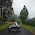Hi
Sir
In example 4 when find register to register delay then not consider setup time but same exaple in STA (3 C) problem 2 when find register to register delay then consider setup time.
plz explain sir
Thank you sir

38.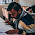hi sir,
first of all thanks for this awesome blog. i have a doubt regarding second question. why haven't u included 2ns delay of buffer in clock path in reg to reg delay? the registers cant be triggered unless clock is being applied to them.

39.Good explanations.
As in example 2, we can generalise, Min clock period = Max data path delay - Minimum clock path delay + Setup time.
In this case for example 5, why are the other data path delays not considered?

40.Sir in example 2
data path max delay= 2+11+2+9+2=26ns,we are adding the wire delay of clock of FF1 to data path instead of clock path.Is there any specific reason for that because I thought it was supposed to be added to the clock path?

41.hello sir, i really loved the way you have explained. but there was a concern that was bothering me from a long time.
Q: if we are calculating max time period from rise edge to fall edge and we are considering all the possible delays including tc2q and setup, then why don't we consider (add) hold time?

42.To calculate max freq, we have to consider 3 types of paths:i/p to o/p, reg to reg and clk port to output port.In example 5, there is no direct path from i/p to o/p, so it is not considered for max freq.Also there is only one path from clk port to output port with delay 2+5+6=13ns.This path should also be considered although it has not max delay.There are 7 reg to reg paths.So, among these 8 paths(7+1),we have to consider the path with max delay.

43.sir why do we consider pin2pin delay while calculating the maximum clock frequency ?
clock does not have any role to play in pin2pin delay containing only combitorial logic circuit